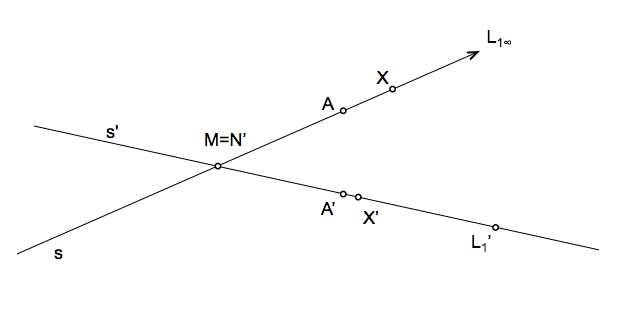# Geometría proyectiva: Determination of homologous elements in series projectiveOne of the first problems we must learn to work in projective geometry is the determination of homologous elements, both beams and series and any provision of bases, or separate superimposed.

To start the study will use the methodology to be used as usual model-based elements “points”, since it is easier to interpret, assuming further that the basis of the corresponding series are separated relate.

Therefore we will consider the determination of homologous elements in two projective series that have common elements. The problem statement, generally, can be:

Given two projective series defined by three pairs of elements (points) counterparts, determine the counterpart of a given point.

The data point can belong to any series and we look therefore belong to the base of the other.

We will solve this problem by using intermediate perspectividades to establish between the two projective series, thereby obtaining the projective axis of the two series (straight and). As we saw, projective axis of the series is the perspective axis of the beams we obtain by projecting the points of a series from any of the other element, and simultaneously project their counterparts from the geometric counterpart vertex element used as the first screening.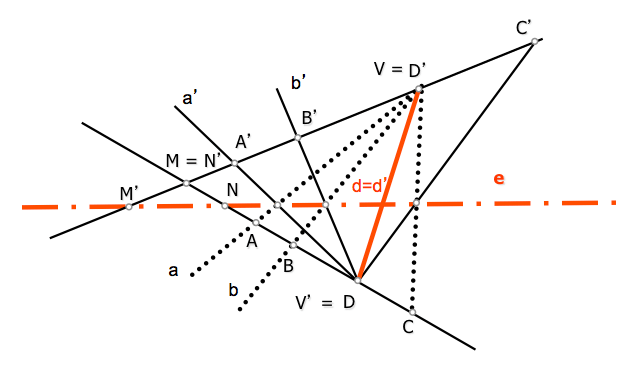Projective axis of two series (Perspectival axis beams)

We will determine in any case, therefore, the projective axis of the series.

### Getting the projective axis of two series:

The different cases that may occur will be determined by the data defining projective series, can be in principle:

• Ordinary pairs of homologous points (3 maximum)
• Limit points of improper points or homologous ( to two possible)
• Homologs of the intersection points of the bases ( 2 maximum)
• Projective axis direction

We can combine these data to determine a specific problem, whenever we bring the necessary number of them. The problem will be determined when we know three pairs of homologous elements or equivalent data. Therefore solve this first case:

Given three points in the series and their counterparts, determine the projective axis of said seriesThe data are the points A, B and C and their corresponding tie points A ', B’ y C’. The intersection point of the base M = N’ contain a point of each of the series.

To determine the projective axis need a couple of points in the same. These can be determined as the intersection of two homologous ray beams perspectival two vertices a pair of homologous points.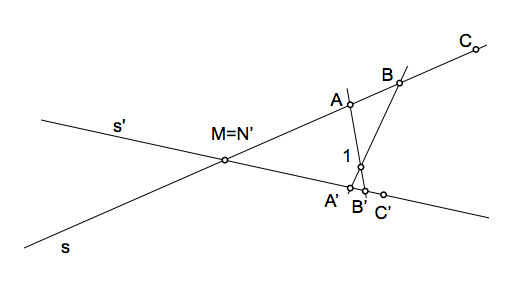Point “1” can be considered as a point of intersection of two homologous ray beams obtained by projecting from A and A’ points B and B ', but we can also understand that the vertices of the beams are B and B’ and the projected points A and A '.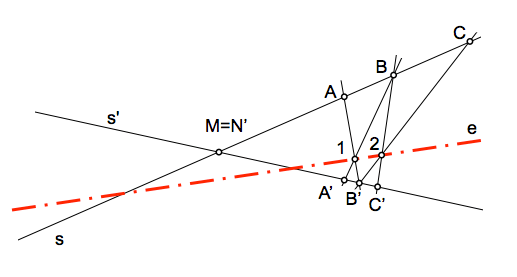The axis has been determined by the previous point and the point “2” which is obtained similarly to the previous, to relate the points B and C with their counterparts B’ y C’.

Homologs of the intersection of the bases are the intersection points of the projection axis with each of the bases. These elements can be obtained like any unknown point X.### Obtaining similar elements

Using the projective axis is easy to determine the counterpart of any point; example we obtain the counterpart of a point X.

To simplify the figure we are left with an element A and its counterpart A'y projective axis series.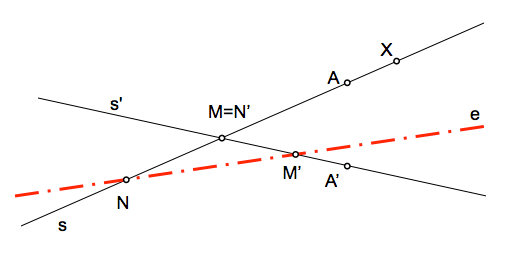If we project from A’ point X, generated lightning and his counterpart (A vertex beam) were cut in the projective axis (point “3”). The ray counterpart contain the element (X’) Searches.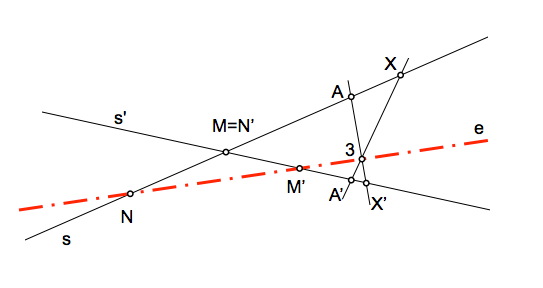### Boundary Elements

Similarly to the case seen for the point X, we can obtain the so-called “limit points” homologs are improper series points (points at infinity). The following figure shows the counterpart of one of them is determined, improper for the s-series. Projecting from the point of the series is limited to obtain the ray parallel to the series that passes through the vertex projection. The intersection of this ray with the projective axis (point 4) allow you to obtain the perspective ray beam counterpart and consequently the point sought.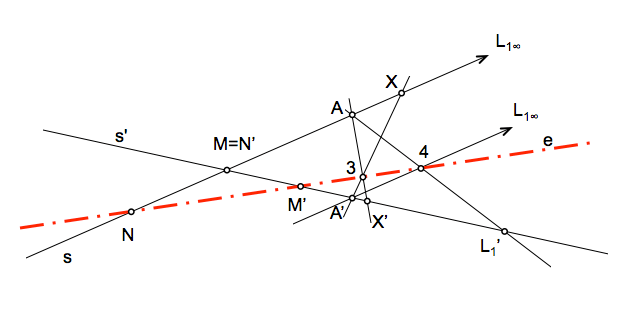### Examples

To complete the study some worked examples that reinforce the concepts proposed.

Determining the projective axis of the series and the homolog of one of the points in the following cases:

to)b)